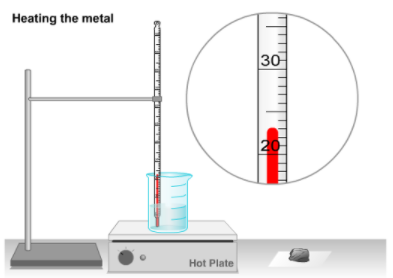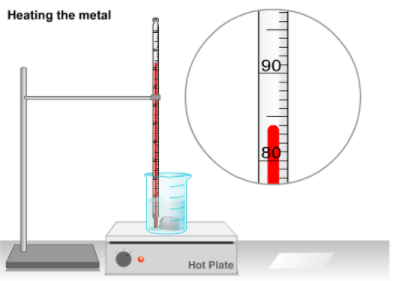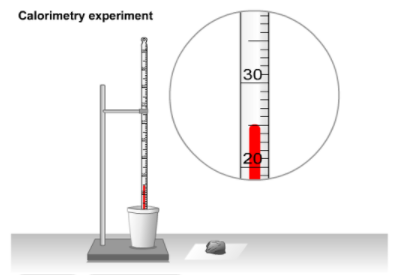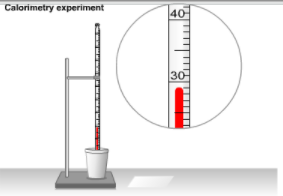# Problem: In this virtual lab you will use a Coffee cup calorimetry to determine the sepecific heat, c, of a metal.  Imagine that, in lab, you record the mass of a piece of metal, mmetal =  45.00 gIn the next step of the lab (as shown in the animation to the left) you heat up the piece of metal. Play the animation, and record the metal's highest temperature, Tmetal:After you heat the metal, the bottom image is the result.In another step of this lab, you record the mass of water (mwater) in a coffee cup as:mwater = 130.00 gWhat is the initial temperature of the water, Twater1, in the coffee cup as shown to the left?Twater1 = In the next step of the lab (as shown in the animation to the left), you place the hot piece of metal into the cool water. Play the animation and record the final temperature of the water, Twater2.Twater2 = With the information above and the specific heat of water, 4.184 J/(g•°C), you calculate the specific heat, c, of the metal.cmetal =

###### FREE Expert Solution

We are being asked to determine the specific heat of the metal.

We will use the following formula to solve this problem:

83% (325 ratings)###### Problem Details

In this virtual lab you will use a Coffee cup calorimetry to determine the sepecific heat, c, of a metal.  Imagine that, in lab, you record the mass of a piece of metal,

mmetal =  45.00 g

In the next step of the lab (as shown in the animation to the left) you heat up the piece of metal. Play the animation, and record the metal's highest temperature, Tmetal:After you heat the metal, the bottom image is the result.In another step of this lab, you record the mass of water (mwater) in a coffee cup as:

mwater = 130.00 g

What is the initial temperature of the water, Twater1, in the coffee cup as shown to the left?Twater1

In the next step of the lab (as shown in the animation to the left), you place the hot piece of metal into the cool water. Play the animation and record the final temperature of the water, Twater2.Twater2

With the information above and the specific heat of water, 4.184 J/(g•°C), you calculate the specific heat, c, of the metal.

cmetal